## 1. Introduction

This article surveys the global distribution of morphological patterns that connect ordinal numerals with cardinal numerals. We associate these two classes of numerals with different functional domains: By cardinal numerals, we understand the set of numerals used in attributive quantification of nouns (e.g. 'four days'). Ordinal numerals typically identify the position a given member of a set occupies relative to other members of the same set (e.g. 'the fourth day'). The main functions of ordinal numerals thus comprise the identification of ranks within a hierarchy and the identification of the temporal order in a sequence of events or the like. For more information on numerals, see Hurford (1975, 1987).

We look at the formal relationship that obtains between cardinal numerals and their ordinal counterparts, i.e. we study morphological and syntactic (periphrastic) ways of deriving ordinals from cardinals. However, we exclude non-morphological marking by word order (cf. §2.2). Furthermore, we restrict our investigation to the first decade (i.e. to the values 1 through 10), because in many languages numeral systems do not go beyond ten, or the use of ordinal numerals is optional with higher numerical values. For the sake of brevity, our examples below list numerals up to value 5. The derivational dependence of ordinal numerals on cardinal numerals is the cover term for regular morphological derivation according to the synchronically valid rules of a given language, and also for all those cases where the morphological relation is of a purely historical nature, so that the word-form of the ordinal numeral reflects obsolete derivational rules of an earlier stage of the same language. Otherwise we consider ordinal numerals derivationally independent of their cardinal counterparts, or in other words, suppletive.

## 2. Defining the values

In Veselinova (1997) and Stolz (2001d), we observed that crosslinguistically the morphological relation of cardinal numerals and ordinal numerals is neither random nor homogeneous. We established eight recurrent patterns whose validity has been corroborated by the present sample of 321 languages. These eight types are listed in the feature-value box:

Values of Map 53A. Ordinal Numerals
ValueRepresentation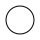Zero: Ordinal numerals do not exist 33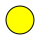One: No distinction of cardinal and ordinal numerals 3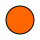First: Cardinal and ordinal numerals are identical except for 'one' and 'first' 12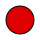One-th: One-th: Ordinal numerals are derived from cardinal numerals 41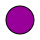First/One-th: All ordinal numerals are derived from cardinal numerals with different allomorphs for 'first' one of which is morphologically independent of 'one' 54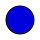Two-th: Two-th: Ordinal numerals from 'two' upwards are derived from cardinal numerals, 'first' is suppletive 110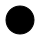Second: 'First' and a small set of consecutive higher ordinal numerals are suppletive 61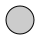Variou-th: Other solutions 7
Total: 321

### 2.1. None

In quite a few languages, ordinal numerals do not exist at all, nor are their functions taken over by cardinal numerals. An example is Kobon (Trans-New Guinea; Davies 1989b: 207-208), where a system of cardinal numerals is attested, but temporal and local adverbs are used instead to encode the relative order of phenomena: ñi nöd  (lit. ‘child before’) ‘first/eldest child’.

### 2.2. One, two, three

What distinguishes this type from the preceding type is the fact that numerals are used to indicate temporal sequence and hierarchical order. Morphologically, there is no way of telling cardinal and ordinal numerals apart, i.e. there is only one multifunctional set of numerals. Sapuan (Bahnaric, Austro-Asiatic; Laos) offers convincing examples of such multifunctional numerals, cf. (1).

(1) Sapuan (Jacq and Sidwell 1999: 30-31)

 a. sɜm bar lăy house two house.class ‘two houses’ b. bar măy law two language Lao ‘my second language is Lao’

In (1a) bar  is used as the equivalent of the cardinal numeral ‘two’, whereas in (1b) it corresponds to the ordinal numeral ‘second’ of the English gloss.

The distinction of cardinal and ordinal functions of numerals via word order alone as attested in Indonesian, can also be seen as an instantiation of the type "One" because the morphology of the numeral itself is not affected in any way by the different syntactic orderings, cf. (2).

(2) Indonesian (Kwee 1981: 97)

 a. gadis ke-tiga girl def-three ‘the third girl’ b. ke-tiga gadis def-three girl ‘the three girls’

If the definite numeral is used in post-nominal position (cf. 2a), its function is clearly that of an ordinal. In pre-nominal position, the numeral can only have a cardinal reading (cf. 2b).

### 2.3. First, two, three

This type shares the identity of cardinal numerals and ordinal numerals with the foregoing type "One, two, three", though with one exception: there is a separate and distinct expression for ‘first’ that is derivationally independent of the cardinal ‘one’. The inventories of ordinal and cardinal numerals proper therefore consist of only one item each. This is the case in Goajiro (Arawakan; Venezuela), where from 2 onwards cardinal and ordinal numerals are identical, cf. (3).

(3) Guajiro (Olza Zubiri and Jusayú 1985: 43-45)

Value

cardinal

ordinal

wanè

pala’jana

pia’ma

apü’nüin

piéinchi

ja’rrái

There is no synchronic or diachronic morphological connection between wanè  ‘one’ and pala’jana  ‘first’. The first position in the numeral paradigm is subject to suppletion.

### 2.4. One-th, two-th, three-th

In this type, cardinal and ordinal numerals are formally distinct and none of the ordinal numerals is derivationally independent of its corresponding cardinal partner. All ordinal numerals are regularly derived from matching cardinal numerals. This general rule extends also to ‘one’ and ‘first’. A typical example of "One-th, two-th, three-th" is Hunzib (Nakh-Daghestanian; eastern Caucasus), cf. (4).

(4) Hunzib (van den Berg 1995: 69-70)

Value

cardinal

ordinal

hə̃s

hə̃s.sə

q’an.u

q’an.u.s

λɑ.nɑ

λɑ.nɑ.s

oq’e.n

oq’e.no.s

λi.no

λi.no.s

The ordinal numerals in this paradigm are derived from the cardinal numerals via suffixation of –s(ə). The phonological shape of this suffix is dependent on the quality of the final segment of the numeral stem. The pair consisting of hə̃s  ‘one’ and hə̃s.sə  ‘first’ behaves morphologically exactly like the rest of the numerals. The paradigm is absolutely regular and free of suppletion.

### 2.5. First/one-th, two-th, three-th

This is a kind of mixture of the two previous types. In principle, regular derivation of ordinal numerals from their corresponding cardinal partners applies on every rank and for every value. However, there are two alternative options for the expression of ‘first’, one following the regular derivation pattern, the other being suppletive. A good example of this allomorphy is Turkish. In this language, every ordinal numeral is derived from its cardinal counterpart via suffixation of –(I)ncI  (the vowel quality changes according to the rules of vowel harmony), cf. (5).

(5) Turkish (Christoph Schröder, p.c.)

Value

cardinal

ordinal

bir

birinci/ilk

iki

ikinci

üç

üçüncü

dört

dörtüncü

beş

beşinci

Accordingly, we find birinci  ‘first’ as a regular derivation of bir  ‘one’. However, alongside this formation there is also the derivationally independent but synonymous form ilk  ‘first’.

### 2.6. First, two-th, three-th

In this type, all ordinal numerals above ‘first’ are regularly derived from their cardinal partners, but ‘first’ is derivationally independent of ‘one’. In Kisi (Southern Atlantic; Guinea) we observe that ordinal numerals are regularly derived from cardinals via the suffix -ndɔɔ, except for tásè  ‘first’, which is derivationally independent of pìlɛɛ  ‘one’, cf. (6).

(6) Kisi (Childs 1995: 112-113, 200-201)

Value

cardinal

ordinal

pìlɛɛ

tásè

mùúŋ

mùúŋndɔɔ

ŋgàá

ŋgàándɔɔ

hìɔɔlú

hìɔɔlúndɔɔ

nùɛɛnú

nùɛɛnúndɔɔ

### 2.7. First, second, three-th

In this type the derivational independence of ordinals is extended further at least to the second rank. In Estonian, for example, alongside the suppletive expression of ‘first’, we also find a derivationally independent form for ‘second’, cf. (7).

(7) Estonian (Lavotha 1973: 52-54)

Value

cardinal

ordinal

üks

esimene

kaks

teine

kolm

kolmas

neli

neljas

viis

viies

The ordinal numeral esimene  ‘first’ has no morphological ties to the cardinal numeral üks  ‘one’, and the same holds of the relation between the ordinal numeral teine  ‘second’ and its cardinal counterpart kaks  ‘two’. The regular derivation of ordinal numerals from cardinal numerals starts with 3 and is achieved via the suffixation of –s.

### 2.8. Various

The eighth group of languages does not form a proper homogeneous type. It is rather the class of individual solutions and exceptions, including a variety of (contact-induced) mixed systems. A pertinent example of an individual solution is Vietnamese, cf. (8).

(8) Vietnamese (Hoàng Thị Chau 1982: 50)

Value

cardinal

ordinal

một

thùʾ nhắt

hai

thùʾ hai

ba

thùʾ ba

bọʾn

thùʾ tuʾ

năm

thùʾ năm

Alongside the suppletive formation of ‘first’, thùʾ nhắt, which is morphologically independent of một  ‘one’, there is also thùʾ tuʾ  ‘fourth’, which has no derivational connection to the cardinal numeral ba  ‘four’. The remaining ordinal numerals are based on their cardinal equivalents. This situation is exceptional insofar as normally suppletion on a value rank n> 1 automatically requires suppletion on the value ranks between n  and 1.

## 3. Geographical distribution

The geographical distribution of the above types is uneven. This asymmetry is most pronounced with "First, second, three-th": of the 61 languages of this type, 47 (= 76%) alone are located in Europe. The most frequently attested type, "First, two-th, three-th", comprises 110 languages on all continents. Of these, 44 (= 40%) are spoken in Africa and 23 (= 21%) in the Americas. The types "One-th, two-th, three-th" and "First/one-th, two-th, three-th" are especially frequent in Asia (14 and 18 languages = 34% and 33% respectively), whereas "None" has two strongholds in the Americas and in the Pacific region with 12 and 17 languages (= 36% and 51% respectively). Asia is the only continent where all of the above types are attested at least once. The types "One-th, two-th, three-th", "First/one-th, two-th, three-th" and "First, two-th, three-th" are attested on all continents, the remaining types being absent from at least one region.